After calculating the core size and wire sizes, it is time to do some physical calculations to see if the right material was chosen. In this part, we will calculate the coil length, the fill of each winding, wire weight, core weights, losses, regulation, and a rough approximation of the DC resistance of the windings.

## Part 3:Physical Design Calculations

### 1. Turns Per Layer

Turns per layer refers to the number magnet wire turns that, when wound, would set side-by-side across the winding area. Calculation for this is dependent upon the use of a tube or bobbin for the winding base structure. A molded plastic bobbin can make for a smaller transformer than a tube. This is due to the bobbin flanges, side walls, that insulate the coil from the core material. Tubes do not have side walls, thereby a margin, or space, between the core and the coil is needed at both ends of the winding. This margin is used as an air gap insolation.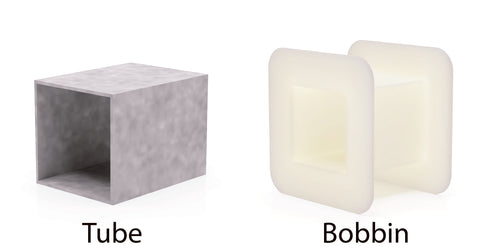The margin width is dependent upon the insolating coating of the magnet wire. Calculating the turns per layer with a modeled plastic bobbin is easier as the bobbin manufacturer supplies the winding length and height. For calculation with a tube, core dimensions, tube dimensions, and experience for the right winding margin gap is needed.

Tube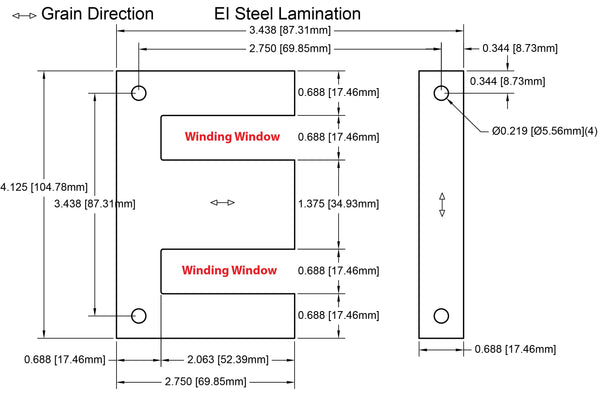First, note the core winding window to find the total winding width and height, listed by the core manufacturer. Next, note the diameter of each of the magnet wire sizes chosen for the design, listed by magnet wire manufacturer. Determine the margin gap needed for each of the magnet wire sizes in the design. Using the voltages, currents, and thermal classification of each magnet wire size chosen, choose a proper air gap/margin size for each winding. UL has several articles and standards for electrical insolation of dry type transformers.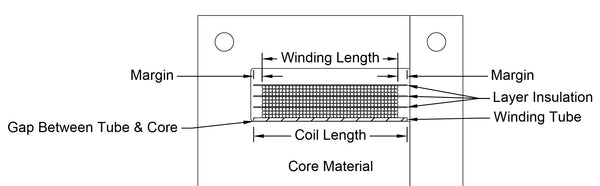The core winding window and required margin are used in the calculation of the winding length across the tube for each winding. To make sure the tube will fit the core winding window, the tube should be at least a 1/16 (0.0625) of an inch smaller than the core winding window width.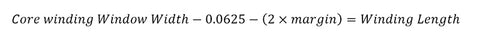Dividing the winding length by the wire diameter will give the turns per layer of the winding. This is an ideal wound turns per layer. Meaning the number of the turns across the winding, if the wound wire is flat and side-by-side with no crossing over or gap between turns.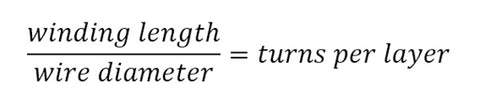Doing side-by-side layer winding with the exact number of turns per layer is not always possible. The speed of the winding machine, smaller the wire size, the tension used on the wire, and even how good the winder is, will determine how well the wire is flat side-by-side wound. A CNC winding machine can help but does not guarantee a flat side-by-side winding, especially with small gauge wire. The speed of the winding machine can affect the tension of the wire. Tension is a crucial factor. Too much tension can stretch wire, making it thinner, and not enough will cause a spongy winding, which can affect coupling and add on to the winding fill.

BobbinWith a molded plastic bobbin, the calculation for the winding length is not needed as the bobbin manufacturer should supply the dimensions. The winding length is the inner distance between the two sides. Calculating the turns per layer utilizes the same formula as for a tube.### 2. Number of Layers Per Winding

To calculate the number of layers per winding, dividing the number of turns (N) of a winding by the number of turns per layer. This will give the number of layers per winding.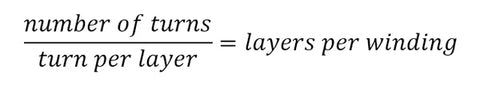Always round up to the next natural number. For instance, if the layers per winding equal 5.4 layers, round up to 6 layers.

### 3. Winding Height

For the winding height, multiply the magnet wire diameter by the number of layers per winding. This will give you the height of each winding.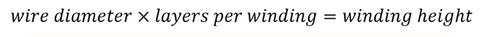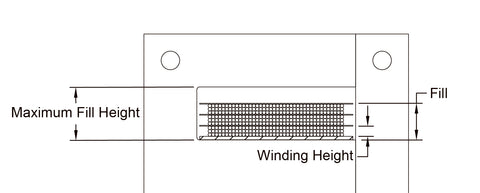### 4. The Fill

The height of each winding is needed to calculate the fill of the transformer. This information along with the thickness of any insolation between the winding layers is used in the calculation. The fill is needed to determine if the coil and any insolation can physically fit in the selected core winding window. Again, if using a tube, additional calculation will have to be performed.

Tube

To calculate the fill for a tube, add up the height of each winding, the thickness of each layer of insolation tape/wrapper used, the thickness of the tube wall, and the distance between the core tongue and the tube wall. There should also be added a distance between the last winding and the core material, for insulation. This distance depends on the rated properties of the material used for the final wrap around the coil. If no insulation or final wrap is used, the distance should be equal or greater than the winding margin gap of the last winding.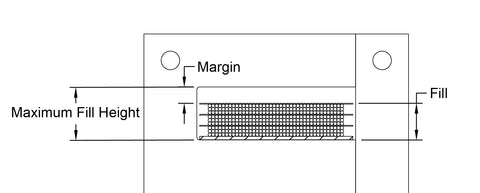The tube thickness will depend on the material and how much support the coil needs. The larger the magnet wire size used, the thicker the tube wall will need to be. Tension and speed when winding will also have an effect. The stronger the tension or a higher the speed can also deform the tube material.

Once you have the total fill height, you can calculate the fill percentage. Divide the total fill height by the winding window height and multiply it by 100.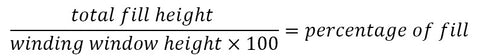Bobbin

For a bobbin, add up the height of each winding, the thickness of each layer of insolation tape/wrapper used. Unlike a tube, the wall thickness or distance between the core and the tube wall are not needed as the inner height of the flange/side of the bobbin is used as the winding window height. This dimension is given by the bobbin manufacturer.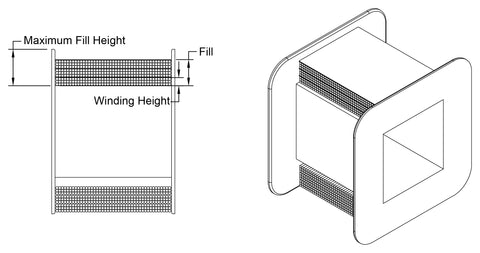The Percentage of Fill should be under 100%. If it is over 100%, then the coil will not physically fit. However, having a Percentage of Fill too low can also have an effect. If the fill percentage is not acceptable, decisions must be made on alterations to the design. Increase the core size will give a larger available winding window. Using a different grade of core material can change the calculated number of turns. Changing the wire size (if possible) can change the DCR of the winding. With some of these changes, a recalculation of the design will need to be done to ensure it is still within the design parameters.

This calculation for the Percentage of Fill is for an ideal side-by-side winding of the coil. This is with the exact number of turns per layer and number of layers per winding for each of the windings. This can be done but can be extremely difficult. With other types of transformers, flat side-by-side winding may not be desirable. This will be discussed more in another section addressing audio transformer designs. For manufacturing, a random winding might be done. Meaning, not perfectly winding side-by-side, having the exact number of turns per layer, and layers per winding. When doing random winding, the Percentage of Fill value will increase depending on the amount of randomness. There are different ways to handle random winding in the design process. Set a percentage that would be consider the winding limit, example, anything over 85% fill value is considered too full. Add a random winding factor in your Percentage of Fill calculation by multiplying the Percentage of Fill by the random winding factor. If you do about 15% random winding, multiply the fill by 1.15.This will increase the Percentage of Fill value by 15% and allows for those times an exact side-by-side, number of turns per layer, or number of layers per winding are not achieved.

Another way is to define a random winding factor for each size of magnet wire. With each transformer design and build, the understanding of random winding techniques and application should be refined.

### 5. The Core

If the turns per layer, layers per winding, and the percentage of fill was calculated for a tube, try going back and do the calculations for a bobbin. The bobbin will have a lower percentage of fill. Using a bobbin may allow for a smaller transformer by increasing the flux density and therefore  needing a smaller core. The temperature rise would need to be checked to determine whether it is within acceptable limits.

Frequencies will also influence the core’s physical size. The lower the operational frequency, the larger the core material will need to be to prevent saturation. For instance, a core would be approximately 10% larger at 50Hz. than at 60Hz. The higher the operational frequency, the smaller the core size can be. Special consideration must be taken if the operational frequency varies, as with an audio transformer. The confederation of the core material should be based on the lowest frequency the transformer is expected to operate at.

### 6. Winding Configuration

At this point a decision of the winding configuration should be made. As the core can determine the lower frequency, coupling between the primary and secondary can determine the high frequency. There are many different winding configurations. Layer Wound is winding of the primary and then the secondary. Layer winding is more common for power transformers or transformers that have a very short operational frequency range. Interleave Wound is the mixing of the primary and secondary windings. The breaking up of the primary and secondary windings and interleaving the windings between one another allowing for better coupling. This is common for audio transformer or transformers with a larger operation frequency range. Filar Wound is winding the primary and secondary side-by-side at the same time. Bi-Filar is two wire winding, Tri-Filar is three wire winding, Quad-Filar is four wire winding, and so forth. Filar winding gives the best coupling and frequency range but is only achievable in some designs. A combination of different winding configurations maybe used. Knowing the best winding configuration for an application is more of an artform that is developed over time and experience.

At this point it is good to draw a diagram and chart of how the transformer will be wound and terminated.By numbering each termination and using the polarity/phase/start dots, an easy chart of the winding order can be done.

### 7. Mean Length

The mean length is used to calculate the winding’s DC resistance (DCR), weight, length, and voltage loss. This is the perimeter of the center of a winding. As each winding is increasingly farther from the center of the bobbin, each winding has its own mean length.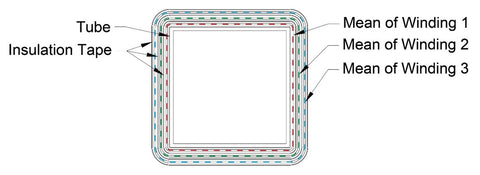By taking the center of a winding, an average of the length of each turn can be determined. With this one can get a rough estimate of the length of magnet wire use for the winding. Any calculations derived from the mean length should be considered rough estimates and not specification worthy. For instance, random winding, parallel windings, taps, tension, etc. will all influence calculations from mean length. The smaller the magnet wire size and/or the more turns on the winding, the more the calculations will be off. It is used to give an idea in the amount of losses and materials needed for manufacturing.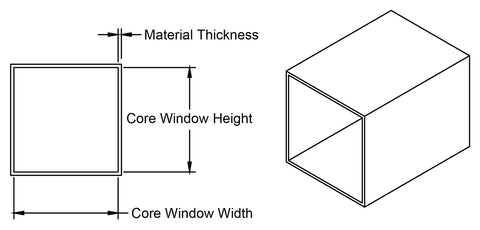You will need the core window width and height, as well as the thickness of the material of the tube or bobbin. Check the manufacturer’s specifications. If you are not using a square tube or bobbin, you can calculate a square core equivalent. This can make the calculations a little easier. To calculate a square core equivalent,3-13 add the core window width multiplied it by 2, and the core window height multiplied by 2, then dividing it by 4.You can also use the called-out core window width and height in the dimensional drawing for your calculations. But, by using the square core window equivalent you can use the same number for the core window width and height.

Take the core window width and add the tube/bobbin material thickness to both ends.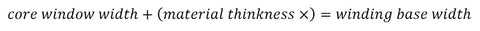Use the same formula to calculate out the winding base height. If you are using a square tube/bobbin or calculated the square core window equivalent. You only need to calculate once, as the width and height are the same.With the winding base width, add the winding height of the first winding, previously calculated. This is the distance from the center of one side of the winding to the center of the other side of the winding. If a square bobbin is used or the square equivalent was calculated, multiply this by 4 to get the mean length of the first winding.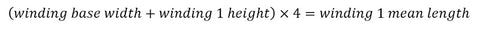If using a rectangular tube or bobbin and did not calculate the square equivalent, calculation for the winding base height must be done. Use the same formula used for the winding base width.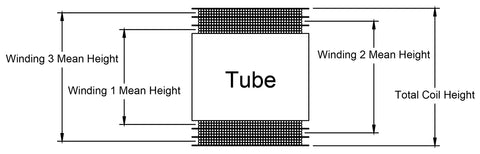First calculate the winding mean width by adding the winding base width to the first winding height. Next calculate the winding mean height by adding the winding base height to the first winding height. Now add the winding mean width to the winding mean height and multiply it by 2 to get the winding mean length. This is the perimeter length of the center of the first winding.Each consecutive winding must also have its mean length calculated. Taking the winding base width and adding any previous winding height and any insolation wrap or tape thickness, using that as the new winding base width for the next winding. Doing the same for the winding base height, if needed. Calculate each winding mean length for each of the windings.

### 8. Magnet Wire Length

The mean winding length is an average length of a turn. Taking the average turn length and multiplying it with the number of turns, we can get a rough estimate length of magnet wire used in the winding.### 9. Winding DC Resistance

Looking at the magnet wire manufacture’s specification and know the length of the magnet wire in the winding, we can get a rough estimate of the DC resistance. The manufacturer’s specifications should note the DC resistance per 1000 feet. This is be done for each of the windings.Again, this is a rough estimate of the DC resistance of the winding.

### 10. Winding Voltage Drop

Start with calculate the voltage drop of the primary. This is simply the DC Resistance of the winding multiplied by the specified current.Use the same formula on each of the secondary windings to calculate each secondary voltage drop.Taking your specified primary voltage and subtract the primary voltage drop, you will get the effective primary input voltage.Using this and the formula for the turn ratio, re-calculate the secondary voltages.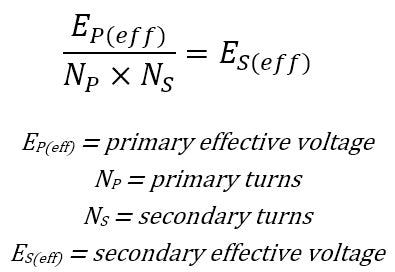By subtracting the secondary voltage drop from the secondary effective voltage an actual voltage is calculated. Again, this is a rough estimate. With this information, adjustments to the design may be needed by changing loss factor. Changing the number of turns, will also change the flux density. Check to make sure that the flux density is within the limits of the core material selected. Full design recalculations should be done again.

### 11. Winding and Coil Weight

There are two ways for calculating the rough weight of each winding. Knowing the DC resistance or the length of the magnet wire used in the winding. The manufacturer should have specification for each magnet wire size on the Ohms per pound and/or feet per pound. Using this information calculate the weight of the magnet wire needed for each windingOnce this is calculated for each winding, you can add them up to get the total weight of the coil and the amount of each magnet wire size needed.

### 12. Core Weight

Core weight is given by the manufacturer. For core that is stacked, like an “E I” core, this is typically the weight of a square core. If the design is not for a square stack, then the weight would need to be calculated.### 13. Losses

Winding Losses
Winding losses are calculated by multiplying the voltage drop by the current. This is done for both the primary and secondary winding.Core Losses
The core manufacture should specify a chart or graph with the core material watts loss per pound. Multiplying the core weight by the watts loss per pound gives the core watts loss.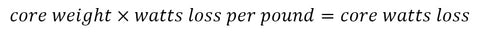Adding up all the winding losses and core loss will give you the total loss. Again, this is a rough estimate.

### 14. Total Weight

Adding all the of the bobbin, windings, insolation, core material, and any hardware, you will get your total weight of the transformer. This information is used to determine rating of the structure that the transformer is being mounted on, whether a PCB or a metal chassis. Some specification may call out a total weight limit. With the previous calculation and knowing what of any hardware and insolation being used, a weight of the transformer can be calculated before building a sample unit.

### 15. Temperature Rise

There are several calculations that can be performed to get the temperature rise for different types of transformers. Temperature rise is the difference between the transformer at ambient temperature to full load. This temperature rise is affected by air flow, confined enclosures, and altitude or depth. For instance, if a transformer is to be used in an airtight box on the ocean floor, it is affected by the still air in the box, the cold water, and the pressure of the depth. With experience, choosing the right materials and flux density would help to achieve this application.

16. Regulation

The voltage measurements of the secondary unloaded, with no load, subtracted by the voltage of the secondary when fully loaded, then divided by fully loaded voltage is the regulation. Multiply this by 100 to get the percentage of regulation.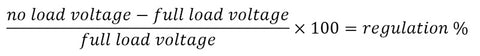If the regulation is called out in the specification, the lower the regulation percentage is desired.

17. Excitation Current

Some specifications call for an excitation current limit. The excitation current is the current needed to drive the core at the proper flux density. If specified, it would need to calculate this out.

The core manufacturer should give values, for the core to be expected at different flux densities. The problem is that these are values under ideal conditions. For these values to work, they would need to modify to for actual working conditions. At this point, it would be a good idea to do a bit more reading on core material selection. As an example, if using M24 22ga. steel core “E I” laminations with a 1 to 1 interleave stack, running at 10000 Gauss, by multiplying the excitation current by 1.5 to one can get a more real-world value. The core manufacturer’s values are a starting point to calculate out the flux density needed for the design to meet the excitation current specifications.

Be aware that there are things that can cause the excitation current not to meet the specifications. Defects in the core material, like laminations that are bent or have large burs on the edges, or a loose core stack can also have a great effect on the excitation current. This is an approximate value. The more experience in designing, the more understanding can be gained of what to expect from core materials.

Coming Soon: Part 4 – Example: Simple Single-Phase Power Transformer Design

Previous: Part 2: Designing a Single-Phase Power Transformer